# estimateMonoCameraFromScene

Estimate monocular camera parameters from scene geometry

Since R2022b

## Syntax

``[pitch,yaw,roll,height,intrinsicsOut] = estimateMonoCameraFromScene(trapezoid,rectangleWidth,rectangleLength,imageSize)``
``[pitch,yaw,roll,height,intrinsicsOut] = estimateMonoCameraFromScene(trapezoid,rectangleWidth,intrinsicsIn)``

## Description

example

````[pitch,yaw,roll,height,intrinsicsOut] = estimateMonoCameraFromScene(trapezoid,rectangleWidth,rectangleLength,imageSize)` estimates the extrinsic and intrinsic parameters of a monocular camera from scene geometry. Specify the pixel coordinates for a trapezoid on the scene image, along with the width and length of the real-world rectangle that corresponds to that trapezoid. The returned extrinsic parameters define the pitch, yaw, and roll rotation angles between the camera coordinate system and vehicle coordinate system axes. The function also returns the height of the camera above the ground, and the intrinsic parameters of the camera `intrinsicsOut`. Use these estimates to configure a `monoCamera` object when a formal calibration with a calibration pattern is not possible. For more information on coordinate systems see, camera coordinate system and vehicle coordinate system.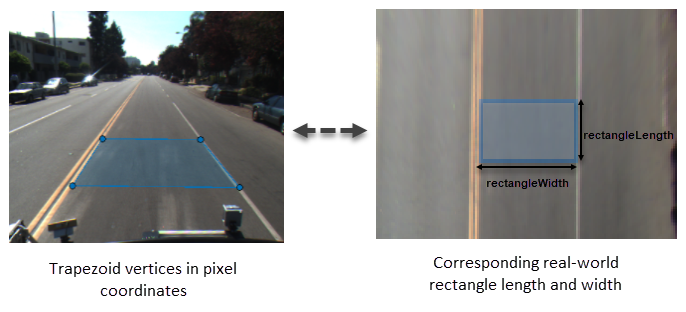```

example

````[pitch,yaw,roll,height,intrinsicsOut] = estimateMonoCameraFromScene(trapezoid,rectangleWidth,intrinsicsIn)` specifies the intrinsic parameters of the camera `intrinsicsIn` instead of the rectangle length and image size. When the camera intrinsic parameters are available, use this syntax to improve the estimates of the extrinsic parameters.```

## Examples

collapse all

Load a MAT file containing a scene image into the workspace.

`load("doubleLaneImageData.mat");`

Specify the pixel coordinates of trapezoid vertices on the scene image that spans the width of two lanes. You must specify the vertices, in the clockwise or counterclockwise order, starting from the upper-left trapezoid vertex.

`trapezoid = [208 456; 170 465; 699 467; 693 456];`

Visualize the trapezoid vertices as annotations on the scene image. Note that the vertices in counterclockwise order.

```doubleLaneImg = insertMarker(doubleLaneImg,trapezoid); text_str = {'UL','LL','LR','UR'}; doubleLaneImg = insertText(doubleLaneImg,trapezoid+[5 5; 5 5; 5 5; 5 -25],text_str); figure imshow(doubleLaneImg)```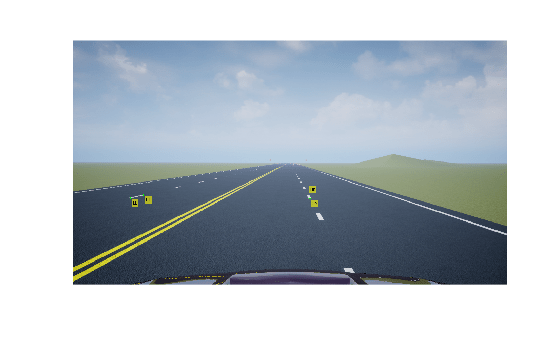Specify the dimensions of the real-world rectangle that corresponds to the trapezoid on the scene image.

```rectangleWidth = 8; rectangleLength = 1.5;```

Specify the size of the image.

`imageSize = size(doubleLaneImg,[1 2]);`

Estimate the extrinsic and intrisic camera parameters by using the `estimateMonoCameraFromScene` function. Display the estimated camera extrinsic and intrinsic parameters.

`[pitch,yaw,roll,height,intrinsics] = estimateMonoCameraFromScene(trapezoid,rectangleWidth,rectangleLength,imageSize)`
```pitch = 0.3032 ```
```yaw = -0.1190 ```
```roll = 2.0457 ```
```height = 1.4508 ```
```intrinsics = cameraIntrinsics with properties: FocalLength: [1.0981e+03 1.0981e+03] PrincipalPoint: [640 360] ImageSize: [720 1280] RadialDistortion: [0 0] TangentialDistortion: [0 0] Skew: 0 K: [3x3 double] ```

Obtain camera extrinsic parameters by specifying the scene geometry interactively on an image when camera intrinsic parameters are available. Then, use the estimated camera parameters to create a `monoCamera` object, and obtain a bird's-eye-view of the scene by using a `birdsEyeView` object.

Obtain Camera Parameters

Load the scene image. Use the known camera intrinsic parameters to create a `cameraIntrinsics` object.

```Im = imread(fullfile(matlabroot, "toolbox", "driving", "drivingdata", "roadSequence", "f00014.png")); focalLength = [309.4362 344.2161]; principalPoint = [318.9034 257.5352]; imageSize = [480 640]; intrinsics = cameraIntrinsics(focalLength,principalPoint,imageSize);```

Display the scene image. Move the four vertices of the trapezoid region of interest (ROI) interactively to specify the desired scene geometry. Then press Enter.

```figure imshow(Im); title("Adjust the trapezoid ROI to select rectangular lane region, press Enter to continue"); vertices = [size(Im,2)/2 size(Im,1)/2;... size(Im,2)/2 size(Im,1)*3/4;... size(Im,2)*3/4 size(Im,1)*3/4;... size(Im,2)*3/4 size(Im,1)/2]; roi = drawpolygon(Position=vertices,FaceAlpha=0.2,LineWidth=1); % Wait until Enter is pressed wasButtonPressed = false; while ~wasButtonPressed wasButtonPressed = waitforbuttonpress; if double(get(gcf,"currentcharacter")) ~= 13 wasButtonPressed = false; end end```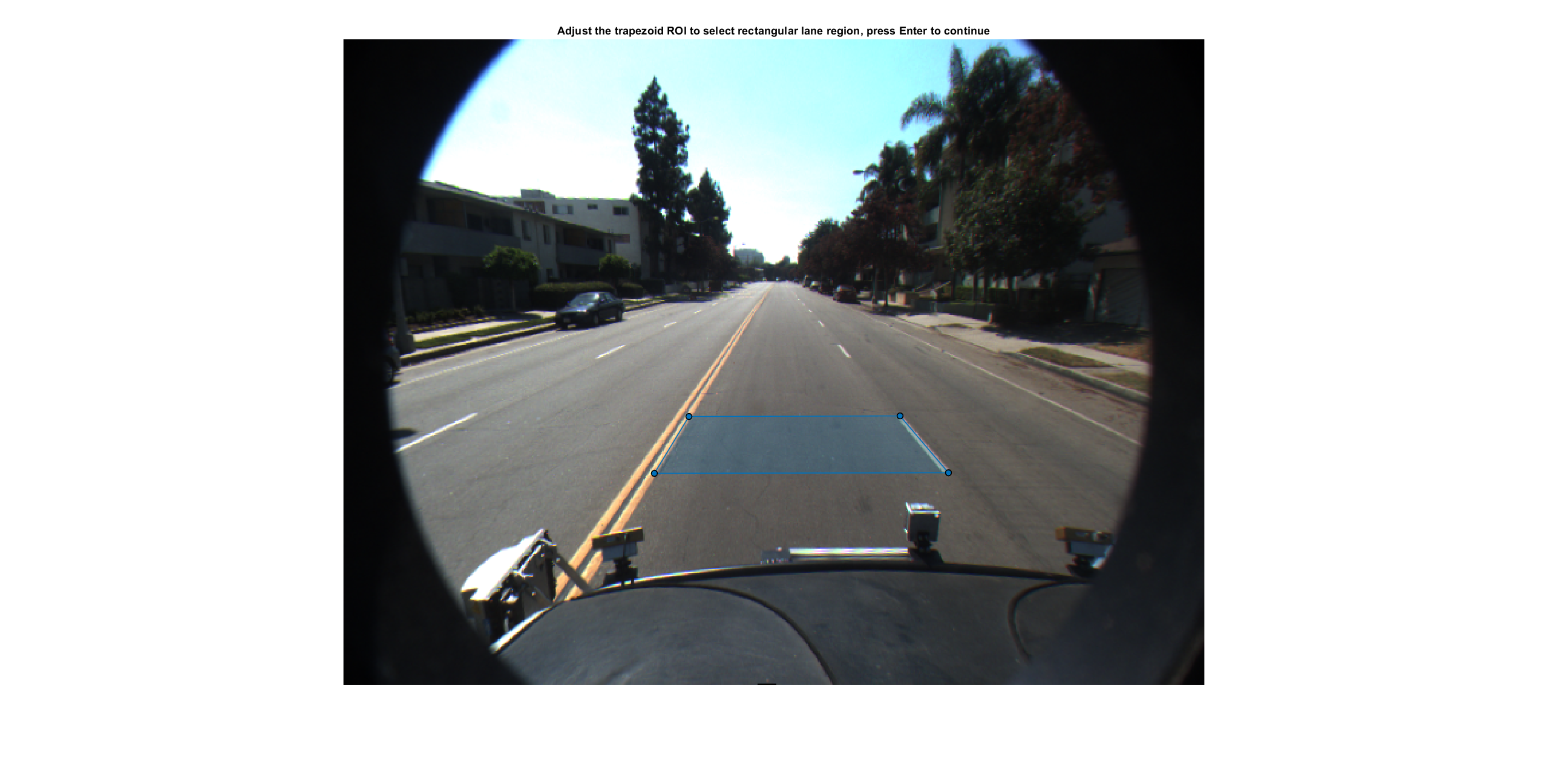Specify the dimensions of the real-world rectangle that corresponds to the trapezoid you specified on the scene image. Because you know the camera intrinsic parameters, you can estimate the extrinsic parameters by specifying only the width of the rectangle.

`rectangleWidth = 3.6;`

Estimate the camera extrinsic parameters to obtain the pitch, roll, yaw, and height of the camera mounted on the vehicle.

`[pitchCal,yawCal,rollCal,heightCal] = estimateMonoCameraFromScene(roi.Position,rectangleWidth,intrinsics);`

Transform Road Image to Bird's-Eye-View Image

Create a `monoCamera` object using the estimated camera extrinsic parameters and the known intrinsic parameters.

`sensor = monoCamera(intrinsics,heightCal,Pitch=pitchCal,Roll=rollCal,Yaw=yawCal);`

Define the area in front of the camera that you want to transform into a bird's-eye view. Specify an area from 3 to 30 meters in front of the camera, with 6 meters to either side of the camera.

```distAhead = 40; spaceToOneSide = 6; bottomOffset = 3; outView = [bottomOffset distAhead -spaceToOneSide spaceToOneSide];```

Set the output image width to 250 pixels. Compute the output height automatically from the width by specifying the length as `NaN`.

`outImageSize = [NaN 250];`

Create a `birdsEyeView` object using the previously defined parameters, and transform the road image. Visualize the bird's-eye-view image.

```birdsEye = birdsEyeView(sensor,outView,outImageSize); BEV = transformImage(birdsEye,Im); imshow(BEV)```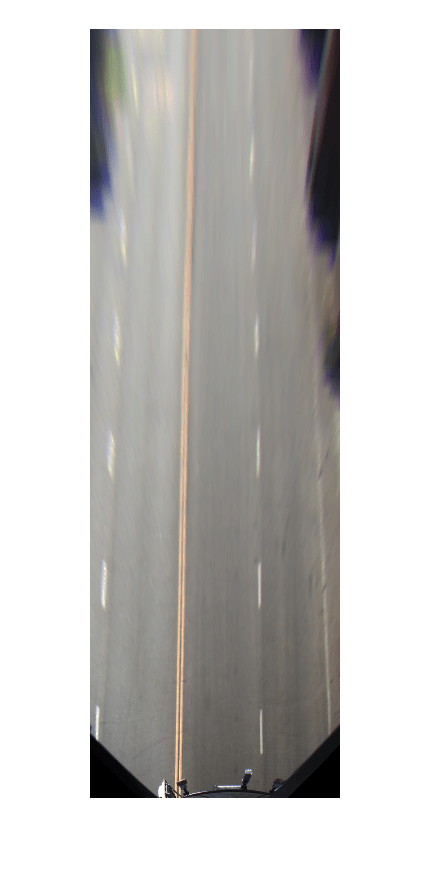## Input Arguments

collapse all

Vertices of a trapezoid on the scene image, in pixel coordinates, specified as a 4-by-2 array. Each row specifies the position of a vertex, and you start with the upper-left vertex of the trapezoid and must follow either clockwise or counterclockwise order.

Data Types: `single` | `double`

Width of the real-world rectangle that corresponds to the trapezoid on the scene image, in meters, specified as a positive scalar.

Data Types: `single` | `double`

Length of the real-world rectangle that corresponds to the trapezoid on the scene image, in meters, specified as a positive scalar.

Data Types: `single` | `double`

Size of the image, in pixels, specified as a two-element vector of the form [m n], where m and n specify the number of rows and columns of pixels, respectively.

Data Types: `single` | `double`

Intrinsic camera parameters, specified as a `cameraIntrinsics` object.

## Output Arguments

collapse all

Pitch angle between the horizontal plane of the vehicle and the optical axis of the camera, returned as a real scalar in degrees. `pitch` uses the ISO convention for rotation, with a clockwise-positive angle direction when looking in the positive direction of the vehicle's YV-axis.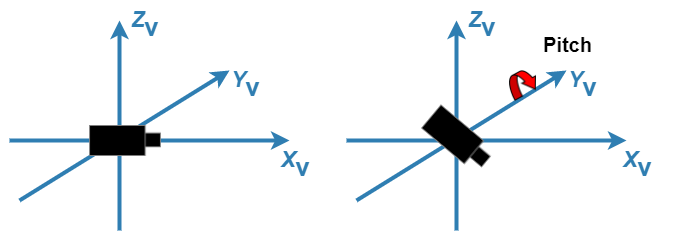For more details, see Angle Directions.

Yaw angle between the XV-axis of the vehicle and the optical axis of the camera, returned as a real scalar in degrees. `yaw` uses the ISO convention for rotation, with a clockwise-positive angle direction when looking in the positive direction of the vehicle's ZV-axis.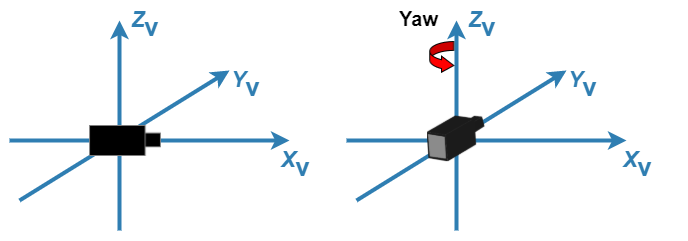For more details, see Angle Directions.

Roll angle of the camera around its optical axis, returned as a real scalar in degrees. `roll` uses the ISO convention for rotation, with a clockwise-positive angle direction when looking in the positive direction of the vehicle's XV-axis.For more details, see Angle Directions.

Perpendicular height from the ground to the focal point of the camera, returned as a nonnegative real scalar in meters.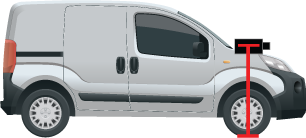Intrinsic camera parameters, returned as a `cameraIntrinsics` object.

#### Limitations

• The `cameraIntrinsics` object returned has the same estimated focal length values in x and y.

• The function does not estimate skew, radial distortion, and tangential distortion. These values are `0` in the returned `cameraIntrinsics` object.

## Version History

Introduced in R2022b